# Flexible Rectangular Beam

Rectangular beam with elastic properties for deformation

Since R2020a

•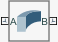Libraries:
Simscape / Multibody / Body Elements / Flexible Bodies / Beams

## Description

The Flexible Rectangular Beam block models a slender beam with a rectangular cross-section that can be solid or hollow. The rectangular beam can have small and linear deformations. These deformations include extension, bending, and torsion. The block calculates the beam cross-sectional properties, such as the axial, flexural, and torsional rigidities, based on the geometry and material properties that you specify.

The geometry of the rectangular beam is an extrusion of its cross-section. The beam cross-section, defined in the xy-plane, is extruded along the z-axis. To define the cross-section, you can specify its dimensions in the Geometry section of the block dialog box. The figure shows a solid beam and a hollow beam. The reference frame is located at the centroid of the beam.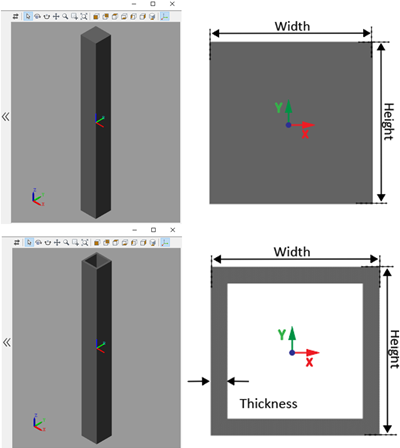This block supports two damping methods and a discretization option to increase the accuracy of the modeling. For more information, see Overview of Flexible Beams.

### Stiffness and Inertia Properties

The block provides two ways to specify the stiffness and inertia properties for a beam. To model a beam made of homogeneous, isotropic, and linearly elastic material, in the Stiffness and Inertia section, set the Type parameter to `Calculate from Geometry`. Then specify the density, Young’s modulus, and Poisson’s ratio or shear modulus. See the Derived Values parameter for more information about the calculated stiffness and inertia properties.

Alternatively, you can manually specify the stiffness and inertia properties, such as flexural rigidity and mass moment of inertia density, by setting the Type parameter to `Custom`. Use this option to model a beam that is made of anisotropic materials. This option decouples the mechanical properties from the beam cross section so you can specify desired mechanical properties without capturing the details of the exact cross section, such as fillet, rounds, chamfers, and tapers.

The manually entered stiffness properties must be calculated with respect to the frame located at the bending centroid. The frame must be in the same orientation as the beam reference frame. The stiffness matrix is

`${\overline{S}}^{b}=\left[\begin{array}{cccc}{S}^{b}& 0& 0& 0\\ 0& {H}_{x}^{b}& -{H}_{xy}^{b}& 0\\ 0& -{H}_{xy}^{b}& {H}_{y}^{b}& 0\\ 0& 0& 0& {H}_{z}^{b}\end{array}\right]$`

where:

• ${S}^{b}$ is the axial stiffness along the beam.

• ${H}_{x}^{b}$ is the centroidal bending stiffness about the x-axis.

• ${H}_{y}^{b}$ is the centroidal bending stiffness about the y-axis.

• ${H}_{z}^{b}$ is the torsional stiffness.

• ${H}_{xy}^{b}$ is the centroidal cross bending stiffness.

The manually entered inertia properties must be calculated with respect to a frame located at the center of mass. The frame must be in the same orientation as the beam reference frame. The mass matrix includes rotatory inertia

`${\overline{M}}^{c}=\left[\begin{array}{cccccc}{m}^{c}& 0& 0& 0& 0& 0\\ 0& {m}^{c}& 0& 0& 0& 0\\ 0& 0& {m}^{c}& 0& 0& 0\\ 0& 0& 0& {i}_{x}^{c}& -{i}_{xy}^{c}& 0\\ 0& 0& 0& -{i}_{xy}^{c}& {i}_{y}^{c}& 0\\ 0& 0& 0& 0& 0& {i}_{z}^{c}\end{array}\right]$`

where:

• ${m}^{c}$ is the mass per unit length.

• ${i}_{x}^{c}$ is the mass moment of inertia density about the x-axis.

• ${i}_{y}^{c}$ is the mass moment of inertia density about the y-axis.

• ${i}_{z}^{c}$ is the polar mass moment of inertia density.

• ${i}_{xy}^{c}$ is the mass product of inertia density.

The beam block uses the classical beam theory where the relation between sectional strains and stress resultants is

`$\left[\begin{array}{c}{\overline{F}}_{z}\\ {\overline{M}}_{x}\\ {\overline{M}}_{y}\\ {\overline{M}}_{z}\end{array}\right]={\overline{S}}^{b}\left[\begin{array}{c}{\overline{\epsilon }}_{z}\\ {\overline{\kappa }}_{x}\\ {\overline{\kappa }}_{y}\\ {\overline{\kappa }}_{z}\end{array}\right]$`

where:

• ${\overline{F}}_{z}$ is the axial force along the beam.

• ${\overline{M}}_{x}$ is the bending moment about the x-axis.

• ${\overline{M}}_{y}$ is the bending moment about the y-axis.

• ${\overline{M}}_{z}$ is the torsional moment about the z-axis.

• ${\overline{\epsilon }}_{z}$ is the axial strain along the beam.

• ${\overline{\kappa }}_{x}$ is the bending curvature about the x-axis.

• ${\overline{\kappa }}_{y}$ is the bending curvature about the y-axis.

• ${\overline{\kappa }}_{z}$ is the torsional twist about the z-axis.

## Ports

### Frame

expand all

Frame by which to connect the beam in a model. In the undeformed configuration, this frame is at half the beam length in the -z direction relative to the origin of the local reference frame.

Frame by which to connect the beam in a model. In the undeformed configuration, this frame is at half the beam length in the +z direction relative to the origin of the local reference frame.

## Parameters

expand all

### Geometry

Choice of solid or hollow cross-section:

• Select `Solid` to model a beam with a solid cross-section.

• Select `Hollow` to model a beam with a hollow cross-section.

Distance between the outer vertical faces of the beam.

Distance between the outer horizontal faces of the beam.

Wall thickness of the hollow cross-section. This parameter specifies the distance between the inner and outer surfaces of the beam.

#### Dependencies

To enable this parameter, set Type to `Hollow`.

Extrusion length of the beam. The beam is modeled by extruding the specified cross-section along the z-axis of the local reference frame. The extrusion is symmetric about the xy-plane, with half of the beam being extruded in the negative direction of the z-axis and half in the positive direction.

### Stiffness and Inertia

Method to use to specify the stiffness and inertia properties, specified as `Calculate from Geometry` or `Custom`.

When you set the parameter to `Calculate from Geometry`, the block calculates the stiffness and inertia properties based on the specified density, Young’s modulus, and Poisson’s ratio or shear modulus. Set the parameter to `Custom` to manually specify the stiffness and inertia properties.

Mass per unit volume of material—assumed here to be distributed uniformly throughout the beam. The default value corresponds to aluminum.

#### Dependencies

To enable this parameter, under the Stiffness and Inertia section, set Type parameter to ```Calculate from Geometry```.

Elastic properties in terms of which to parameterize the beam. These properties are commonly available from materials databases.

#### Dependencies

To enable this parameter, under the Stiffness and Inertia section, set Type parameter to ```Calculate from Geometry```.

Young's modulus of elasticity of the beam. The greater its value, the stronger the resistance to bending and axial deformation. The default value corresponds to aluminum.

#### Dependencies

To enable this parameter, under the Stiffness and Inertia section, set Type parameter to ```Calculate from Geometry```.

Poisson's ratio of the beam. The value specified must be greater than or equal to `0` and smaller than `0.5`. The default value corresponds to aluminum.

#### Dependencies

To enable this parameter, under the Stiffness and Inertia section, set Type parameter to ```Calculate from Geometry``` and set the Specify parameter to Young's Modulus and Poisson's Ratio.

Shear modulus (or modulus of rigidity) of the beam. The greater its value, the stronger the resistance to torsional deformation. The default value corresponds to aluminum.

#### Dependencies

To enable this parameter, under the Stiffness and Inertia section, set Type parameter to ```Calculate from Geometry``` and set the Specify parameter to Young's and Shear Modulus.

Calculated values of the mass and stiffness sectional properties of the beam. Click Update to calculate and display those values.

The properties given include Centroid and Shear Center. The centroid is the point at which an axial force extends (or contracts) the beam without bending. The shear center is that through which a transverse force must pass to bend the beam without twisting.

The stiffness sectional properties are computed as follows:

• Axial Rigidity: EA

• Flexural Rigidity: [EIx, EIy]

• Cross Flexural Rigidity: EIxy

• Torsional Rigidity: GJ

The mass sectional properties are computed as follows:

• Mass per Unit Length: ρA

• Mass Moment of Inertia Density: [ρIx, ρIy]

• Mass Product of Inertia Density: ρIxy

• Polar Mass Moment of Inertia Density: ρIp

The equation parameters include:

• A — Cross-sectional area

• ρ — Density

• E — Young's modulus

• G — Shear modulus

• J — Torsional constant (obtained from the solution of Saint-Venant's warping partial differential equation)

The remaining parameters are the relevant moments of area of the beam. These are calculated about the axes of a centroidal frame—one aligned with the local reference frame but located with its origin at the centroid. The moments of area are:

• Ix, Iy — Centroidal second moments of area:

$\left[{I}_{x},{I}_{y}\right]=\left[\underset{A}{\int }{\left(y-{y}_{c}\right)}^{2}dA,\underset{A}{\int }{\left(x-{x}_{c}\right)}^{2}dA\right]$,

• Ixy — Centroidal product moment of area:

${I}_{xy}=\underset{A}{\int }\left(x-{x}_{c}\right)\left(y-{y}_{c}\right)dA$,

• Ip — Centroidal polar moment of area:

${I}_{P}={I}_{x}+{I}_{y}$,

where xc and yc are the coordinates of the centroid.

Centroid of the beam cross section, specified as a 2-by-1 array. The array specifies the x and y coordinates of the centroid with respect to the beam reference frame.

#### Dependencies

To enable this parameter, in the Stiffness and Inertia section, set Type to `Custom`.

Intersection of the neutral axis and cross section, specified as a 2-by-1 array. The array specifies the x and y coordinates of the centroid with respect to the beam reference frame.

#### Dependencies

To enable this parameter, in the Stiffness and Inertia section, set Type to `Custom`.

Beam shear center, specified as a 2-by-1 array. The array specifies the x and y coordinates of the point with respect to the beam reference frame.

#### Dependencies

To enable this parameter, in the Stiffness and Inertia section, set Type to `Custom`.

Resistance against the deformation along the beam longitudinal direction, specified as a scalar. This parameter specifies the ${S}^{b}$ element of the stiffness matrix.

#### Dependencies

To enable this parameter, in the Stiffness and Inertia section, set Type to `Custom`.

Resistance against bending deformations, specified as a 2-by-1 array. The array specifies the ${H}_{x}^{b}$ and ${H}_{y}^{b}$ elements of the stiffness matrix.

#### Dependencies

To enable this parameter, in the Stiffness and Inertia section, set Type to `Custom`.

Resistance against the bending deformation about the x-axis in response to bending moment about the y-axis, specified as a scalar. This parameter specifies the ${H}_{xy}^{b}$ element of the stiffness matrix.

#### Dependencies

To enable this parameter, in the Stiffness and Inertia section, set Type to `Custom`.

Resistance against twisting about the longitudinal axis of the beam, specified as a scalar. This parameter specifies the ${H}_{z}^{b}$ element of the stiffness matrix.

#### Dependencies

To enable this parameter, in the Stiffness and Inertia section, set Type to `Custom`.

Center of mass, specified as a 2-by-1 array. The array specifies the x and y coordinates of the point with respect to the beam reference frame.

#### Dependencies

To enable this parameter, in the Stiffness and Inertia section, set Type to `Custom`.

Mass per unit length, specified as a scalar. This parameter specifies the ${m}^{c}$ element of the mass matrix.

#### Dependencies

To enable this parameter, in the Stiffness and Inertia section, set Type to `Custom`.

Resistance against rotation about the x and y axes, specified as a 2-by-1 array. The array specifies the ${i}_{x}^{c}$ and ${i}_{y}^{c}$ elements of the mass matrix.

#### Dependencies

To enable this parameter, in the Stiffness and Inertia section, set Type to `Custom`.

Resistance against rotation about the x-axis due to the moment about the y-axis, specified as a scalar. This parameter specifies the ${i}_{xy}^{c}$ element of the mass matrix.

#### Dependencies

To enable this parameter, in the Stiffness and Inertia section, set Type to `Custom`.

### Damping

Damping method to apply to the beam:

• Select `None` to model undamped beams.

• Select `Proportional` to apply the proportional (or Rayleigh) damping method. This method defines the damping matrix [C] as a linear combination of the mass matrix [M] and stiffness matrix [K]:

$\left[C\right]=\alpha \left[M\right]+\beta \left[K\right]$,

where α and β are the scalar coefficients.

• Select `Uniform Modal` to apply the uniform modal damping method. This method applies a single damping ratio to all the vibration modes of the beam. The larger the value, the faster vibrations decay.

Coefficient, α, of the mass matrix. This parameter defines damping proportional to the mass matrix [M].

#### Dependencies

To enable this parameter, set Type to `Proportional`.

Coefficient, β, of the stiffness matrix. This parameter defines damping proportional to the stiffness matrix [K].

#### Dependencies

To enable this parameter, set Type to `Proportional`.

Damping ratio, ζ, applied to all beam vibration modes in the uniform modal damping model. The larger the value, the faster beam vibrations decay.

• Use ζ = 0 to model undamped beams.

• Use ζ < 1 to model underdamped beams.

• Use ζ = 1 to model critically damped beams.

• Use ζ > 1 to model overdamped beams.

#### Dependencies

To enable this parameter, set Type to ```Uniform Modal```.

Data Types: `double`

### Discretization

Number of finite elements in the beam model. Increasing the number of elements always improves accuracy of the simulation. But practically, at some point, the increase in accuracy is negligible when there are many elements. Additionally, a higher number of elements increases the computational cost and slows down the speed of the simulation.

### Fidelity

Method to use to model flexible bodies, specified as `None` or ```Modally Reduced```. Set the parameter to `None` to use full nodal elastic coordinates generated by the finite-element method or set the parameter to `Modally Reduced` to use the modal transformation method to reduce the elastic coordinates of the body. For both settings, the block uses the floating frame of the reference formulation [1-2] to couple the body with its elastic deformation.

Retained modes, specified as an integer in range [0, n], where n is the number of elastic degrees of freedom of the body. If you set the number to 0 the flexible body is treated as a rigid body.

#### Dependencies

To enable this parameter, set Reduction to ```Modally Reduced```.

### Graphic

Type of the visual representation of the beam, specified as `From Geometry` or `None`. Set the parameter to `From Geometry` to show the visual representation of the beam. Set the parameter to `None` to hide the beam in the model visualization.

Parameterizations for specifying visual properties. Select `Simple` to specify Color and Opacity. Select `Advanced` to specify more visual properties, such as Specular Color, Ambient Color, Emissive Color, and Shininess.

#### Dependencies

To enable this parameter, set Type to `From Geometry`.

Color of the graphic under direct white light, specified as an [R G B] or [R G B A] vector on a 0–1 scale. An optional fourth element (A) specifies the color opacity on a scale of 0–1. Omitting the opacity element is equivalent to specifying a value of 1.

#### Dependencies

To enable this parameter, set:

1. Type to `From Geometry`

2. Visual Properties to `Simple`

Graphic opacity, specified as a scalar in the range of 0 to 1. A scalar of 0 corresponds to completely transparent, and a scalar of 1 corresponds to completely opaque.

#### Dependencies

To enable this parameter, set:

1. Type to `From Geometry`

2. Visual Properties to `Simple`

Color of the light due to diffuse reflection, specified as an [R,G,B] or [R,G,B,A] vector with values in the range of 0 to 1. The vector can be a row or column vector. The optional fourth element specifies the color opacity. Omitting the opacity element is equivalent to specifying a value of 1.

The diffuse color reflects the main color of the rendered beam and provides shading that gives the rendered object a three-dimensional appearance.

#### Dependencies

To enable this parameter, set:

1. Type to `From Geometry`

2. Visual Properties to `Advanced`

Color of the light due to specular reflection, specified as an [R,G,B] or [R,G,B,A] vector with values in the range of 0 to 1. The vector can be a row or column vector. The optional fourth element specifies the color opacity. Omitting the opacity element is equivalent to specifying a value of 1. This parameter changes the color of the specular highlight, which is the bright spot on the rendered beam due to the reflection of the light from the light source.

#### Dependencies

To enable this parameter, set:

1. Type to `From Geometry`

2. Visual Properties to `Advanced`

Color of the ambient light, specified as an [R,G,B] or [R,G,B,A] vector with values in the range of 0 to 1. The vector can be a row or column vector. The optional fourth element specifies the color opacity. Omitting the opacity element is equivalent to specifying a value of 1.

Ambient light refers to a general level of illumination that does not come directly from a light source. The Ambient light consists of light that has been reflected and re-reflected so many times that it is no longer coming from any particular direction. You can adjust this parameter to change the shadow color of the rendered beam.

#### Dependencies

To enable this parameter, set:

1. Type to `From Geometry`

2. Visual Properties to `Advanced`

Color due to self illumination, specified as an [R,G,B] or [R,G,B,A] vector in the range of 0 to 1. The vector can be a row or column vector. The optional fourth element specifies the color opacity. Omitting the opacity element is equivalent to specifying a value of 1.

The emission color is color that does not come from any external source, and therefore seems to be emitted by the beam itself. When a beam has a emissive color, the beam can be seen even if there is no external light source.

#### Dependencies

To enable this parameter, set:

1. Type to `From Geometry`

2. Visual Properties to `Advanced`

Sharpness of specular light reflections, specified as a scalar number on a 0–128 scale. Increase the shininess value for smaller but sharper highlights. Decrease the value for larger but smoother highlights.

#### Dependencies

To enable this parameter, set:

1. Type to `From Geometry`

2. Visual Properties to `Advanced`

### Frames

Select to expose the A port.

Select to expose the B port.

Click the Create button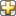to open a pane for creating a new body-attached frame. In this pane, you can specify the name, origin, and orientation for the frame.

• To name the custom frame, click the text field of the Frame Name parameter. The name identifies the corresponding port on the beam block and in the tree view pane of the Mechanics Explorer.

• To select the Frame Origin for the custom frame, use one of the following methods:

• At Reference Frame Origin: Make the new frame origin coincident with the origin of the reference frame of the undeformed beam.

• Based on Geometric Feature: Make the new frame origin coincident with the center of the selected undeformed geometry feature. Valid features include surfaces, lines, and points. Select a feature from the visualization pane, then click Use Selected Feature to confirm the location of the origin. The name of the origin location appears in the field below this option.

• To define the orientation of the custom frame, under the Frame Axes section, select the Primary Axis and Secondary Axis of the custom frame and then specify their directions.

Use the following methods to select a vector for specifying the directions of the primary and secondary axes. The primary axis is parallel to the selected vector and constrains the remaining two axes to its normal plane. The secondary axis is parallel to the projection of the selected vector onto the normal plane.

• Along Reference Frame Axis: Selects an axis of the reference frame of the undeformed beam.

• Based on Geometric Feature: Selects the vector associated with the chosen geometry feature of the undeformed beam. Valid features include surfaces and lines. The corresponding vector is indicated by a white arrow in the visualization pane. You can select a feature from the visualization pane and then click Use Selected Feature to confirm the selection. The name of the selected feature appears in the field below this option.

Frames that you have created. `N` is a unique identifying number for each custom frame.

• Click the text field to edit the name of an existing custom frame.

• Click the Edit button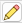to edit other aspects of the custom frame, such as origin and axes.

• Click the Delete button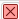to delete the custom frame.

#### Dependencies

To enable this parameter, create a frame by clicking New Frame.

 Shabana, Ahmed A. Dynamics of Multibody Systems. Fourth edition. New York: Cambridge University Press, 2014.

 Agrawal, Om P., and Ahmed A. Shabana. “Dynamic Analysis of Multibody Systems Using Component Modes.” Computers & Structures 21, no. 6 (January 1985): 1303–12. https://doi.org/10.1016/0045-7949(85)90184-1.